For this document, I will be focusing on predicing the type of seismic event based on the other variables in the USGS data set.

As in the EDA, only variables which measure the events themselves will be used. Additionally, we will restrict our sample to events occurring within the continental United States.

# Data shaping

## Subsetting and Clean-Up

library(dplyr, warn.conflicts = FALSE)
library(tidyr, , warn.conflicts = FALSE)
library(ggplot2)
seismic <- seismic %>%
dplyr::select(-magType, -nst, -gap, -dmin, -net, -updated) %>%
mutate(type = factor(type)) %>%
filter(latitude < 49,
latitude > 18,
longitude < -63.37,
longitude > -124.6) %>%
dplyr::select(type, mag, depth, rms) %>%
na.omit()
summary(seismic)
##                   type           mag            depth
##  anthropogenic event:   4   Min.   :1.000   Min.   : -3.450
##  earthquake         :8546   1st Qu.:1.210   1st Qu.:  3.180
##  explosion          :  85   Median :1.520   Median :  6.727
##  landslide          :   1   Mean   :1.759   Mean   :  9.063
##  mining explosion   :  14   3rd Qu.:2.185   3rd Qu.:  9.810
##  quarry blast       : 209   Max.   :6.200   Max.   :182.000
##       rms
##  Min.   :0.0000
##  1st Qu.:0.0800
##  Median :0.1500
##  Mean   :0.1975
##  3rd Qu.:0.2400
##  Max.   :2.3500

Notice that we have only one landslide, which means we won’t be able to train a multinomial model to predict it and still have one in the testing set, so we should remove the observation.

Additionally, we have some very small cells, which also tends to cause problems in multinomial prediction problems. Because of this, we’ll collapse mining explosions and anthropogenic events into the explosions class (operating under the assumption that the anthropenic events are explosions).

seismic <- seismic %>% filter(type != "landslide") %>%
mutate(type = factor(type))
seismic$type <- factor(ifelse(seismic$type == "anthropogenic event" |
seismic$type == "mining explosion", "explosion", as.character(seismic$type)))
seismic %>% dplyr::select(type) %>% summary()
##            type
##  earthquake  :8546
##  explosion   : 103
##  quarry blast: 209

As a reminder, definitions of the covariates can be found here.

## Preliminary Analysis

To start, let’s examine the proportions of each event by each of our covariates.

prop.tab.mag <- with(seismic,
data.frame(prop.table(table(type, cut(mag, 4)))))
prop.tab.depth <- with(seismic,
data.frame(prop.table(table(type, cut(depth, 4)))))
prop.tab.rms <- with(seismic,
data.frame(prop.table(table(type, cut(rms, 4)))))
prop.tab <- data.frame(rbind(prop.tab.mag, prop.tab.depth,
prop.tab.rms),
var = rep(c("magnitude", "depth", "rms"),
each = 12))
colnames(prop.tab) <- c("type", "range", "prop", "var")
ggplot(prop.tab, aes(x=range, y=prop, color=type, group=type)) +
geom_line() + facet_wrap(~var, ncol = 3, scale = "free_x") +
xlab("Ranges") + ylab("Proportion") +
ggtitle("Proportions of Event Types by Covariates") +
theme(legend.position = "top")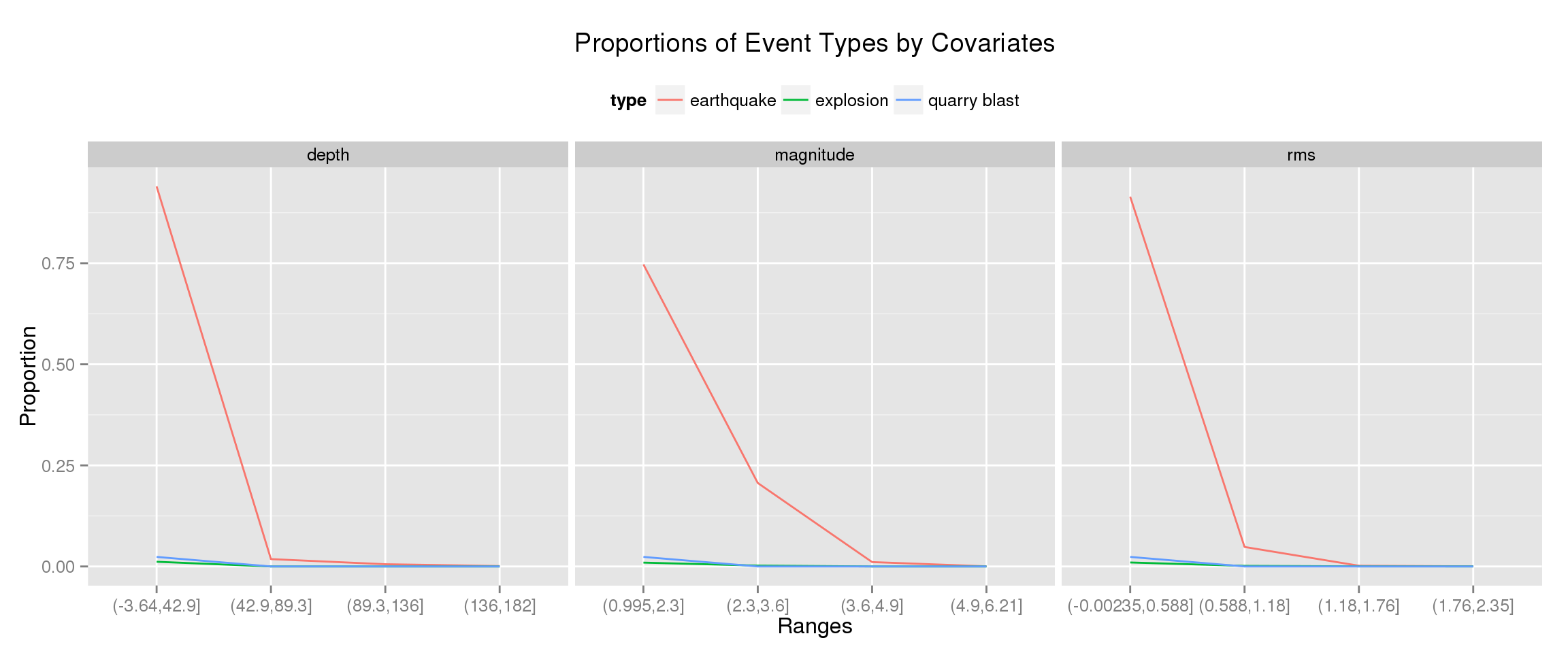From these plots, we can see that predicting is going to be difficult. Almost all events are low-magnitude, surface level earthquakes which had well-predicted velocities.

Explosions and quarry plots have some differerence at the low end of the scales, but differentating between them becomes more difficult as we increase all three measures.

## Creating the Testing and Training Sets

From here we scale and center our variables and set up a training and testing sets. Note because of the diproportionate number of events, we’ll go with a stratified approach over a simple random sample of rows, otherwise we might end up with a training set composed entirely of earthquakes. Because some of the R packages don’t accept model formulas, we also need to split up each set into the response and the features.

seismic[, -1] <- scale(seismic[, -1])
set.seed(100)
sampl <-  c(
(data.frame(which(seismic$type == "earthquake")) %>% sample_frac(0.8))[,1], (data.frame(which(seismic$type == "explosion")) %>%
sample_frac(0.8))[,1],
(data.frame(which(seismic$type == "quarry blast")) %>% sample_frac(0.8))[,1]) seismic.train <- seismic[sampl,] seismic.test <- seismic[-sampl,] x.train <- as.matrix(seismic.train[,-1]) y.train <- seismic.train[,1] x.test <- as.matrix(seismic.test[,-1]) y.test <- seismic.test[,1] # Modelling ## Multinomial Regression As a baseline, we’ll start with basic multinomial logit model. library(nnet) (seismic.multinom <- multinom(type ~ depth + mag + rms, seismic.train)) ## # weights: 15 (8 variable) ## initial value 7784.766678 ## iter 10 value 687.759231 ## iter 20 value 600.728069 ## final value 600.586206 ## converged ## Call: ## multinom(formula = type ~ depth + mag + rms, data = seismic.train) ## ## Coefficients: ## (Intercept) depth mag rms ## explosion -15.62139 -19.74072 0.1548999 0.5176571 ## quarry blast -18.63146 -24.75921 -0.5934056 -0.8815323 ## ## Residual Deviance: 1201.172 ## AIC: 1217.172 We see that, for both explosions and quarry blasts, depth decreases the likelihood of that type occuring. Because we have so many low magnitude earthquakes (as per the Gutenberg–Richter law), increasing the magnitude actually increases the chance of an event being an explosion. It also appears that the velocities of explosions are harder to estimate, so a higher rms is also associated with explosions. Both relationships in explosions are reversed for quarry blast’s. Increasing the magnitude and the rms decrease the likelihood of an event being a quarry blast over an earthquake. Let’s see how the predictions panned out. library(caret, warn.conflicts = FALSE) ## Loading required package: lattice library(e1071) multinom.pred <- predict(seismic.multinom, newdata = seismic.test, type = "class") err <- confusionMatrix(multinom.pred, y.test) err$table
##               Reference
## Prediction     earthquake explosion quarry blast
##   earthquake         1704        18           42
##   explosion             1         3            0
##   quarry blast          4         0            0
err$overall["Accuracy"] ## Accuracy ## 0.9633183 The multinomial model only miss-classified five out of 1709 earthquakes in the testing set. The other event types where less successful, however. Eighteen of twenty-one explosions and all quarry blasts were miss-classified as earthquakes. On the positive side, the model never confused these event types. The overall classification accuracy was $$96.33\%$$. This may seem like a relatively good measure until we consider that would get an accuracy rate of $$96.44\%$$ if we simply guess that every event is an earthquake (i.e., $$1709$$ earthquakes $$\div 1772$$ total events = $$0.9644$$). ## Regularization Methods Since the multinomial logit model did more poorly than simply guessing that every event is an earthquake, we may want to see if a regularization method can do better. These methods are typically used with highly correlated covariates (e.g., the lasso) or in high-dimensional space (e.g., ridge regression). While we have neither problem here, biasing our covariance matrix may still lead to more accurate classification. First, we’ll create a prettier plot for examining the $$\lambda$$ values against the fit. ggnet <- function(x){ cv.obj <- x dat <- data.frame(lambda = cv.obj$lambda, dev = cv.obj$cvm, ymax = cv.obj$cvup, ymin = cv.obj$cvlo) xints <- log(c(cv.obj$lambda.min, cv.obj$lambda.1se)) ggplot(dat, aes(x = log(lambda), y = dev)) + geom_point(color = "darkred") + geom_errorbar(aes(ymax = ymax, ymin= ymin), color = "darkgrey") + geom_vline(xintercept = xints, linetype = "longdash") + ylab("Multinomial Deviance") } ### Multinomial Ridge Regression To start, we’ll apply a ridge penalty to the model, which is equivalent to an elastic net with $$\alpha = 0$$. library(glmnet, warn.conflicts = FALSE) ## Loading required package: Matrix ## ## Attaching package: 'Matrix' ## ## The following object is masked from 'package:tidyr': ## ## expand ## ## Loaded glmnet 1.9-8 library(doMC, warn.conflicts = FALSE) ## Loading required package: foreach ## Loading required package: iterators ## Loading required package: parallel registerDoMC(cores = 6) seismic.ridge <- cv.glmnet(x.train, y.train, family = "multinomial", alpha = 0, parallel = TRUE) seismic.ridge$lambda.1se
##  0.003556561
ggnet(seismic.ridge)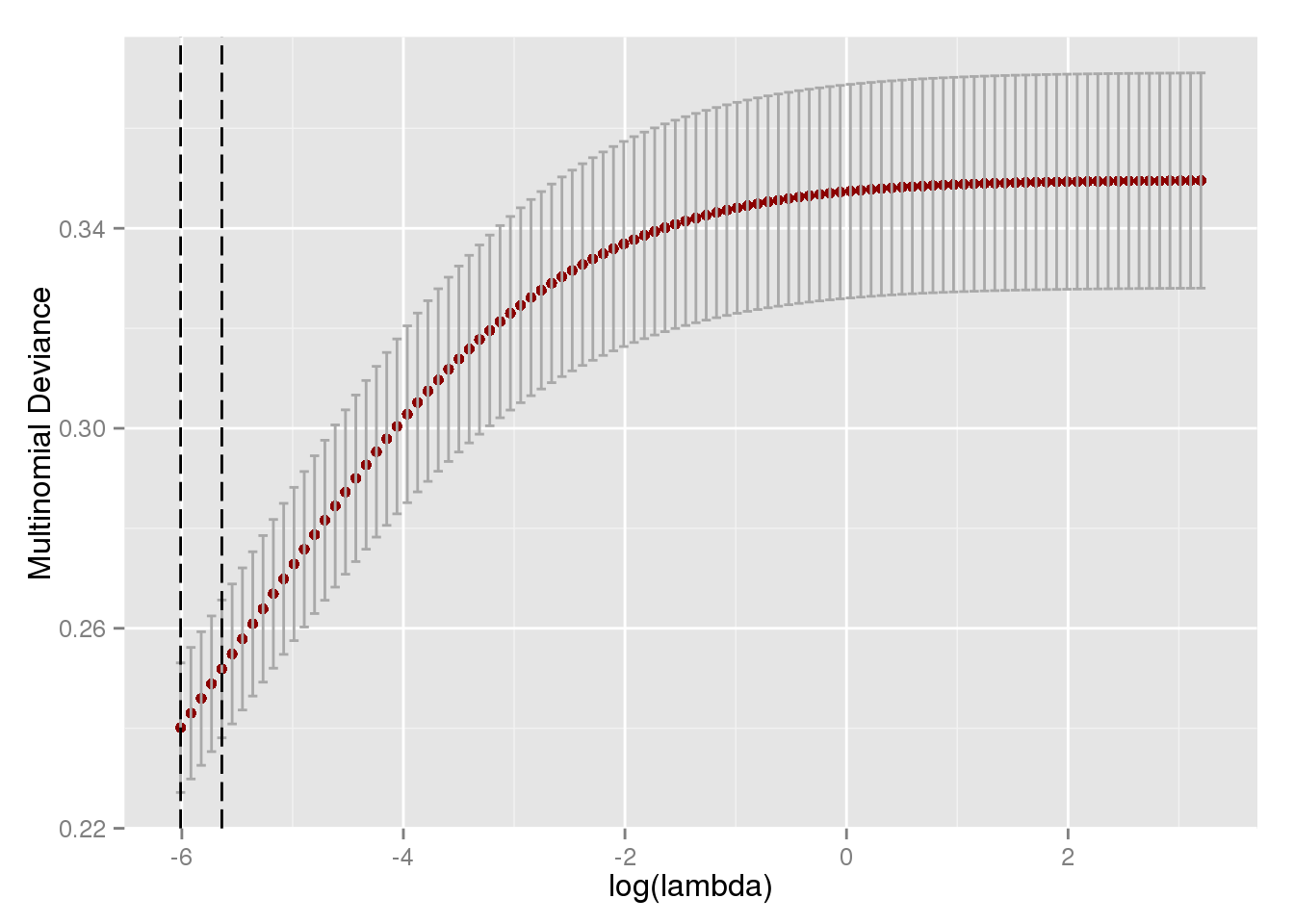We can see the deviance increases rapidly as we increase $$\lambda$$, suggesting that the ridge penalty isn’t doing much for the fit this data set.

ridge.pred <- factor(predict(seismic.ridge, newx = x.test, type = "class"))
levels(ridge.pred) <- levels(y.test)
err.ridge <- confusionMatrix(ridge.pred, y.test)
err.ridge$table ## Reference ## Prediction earthquake explosion quarry blast ## earthquake 1709 21 42 ## explosion 0 0 0 ## quarry blast 0 0 0 err.ridge$overall["Accuracy"]
## Accuracy
## 0.964447

While the multinomial GLM saw a decrease in its accuracy because it incorrectly classified more earthquakes than correctly classified the other types, the ridge model simply failed to distinguish between the event types.

### Lasso

Instead of using a ridge penalty, we might try a lasso penalty (equivalent to the elastic net with $$\alpha = 1$$.

seismic.lasso <- cv.glmnet(x.train, y.train, family = "multinomial",
alpha = 1, parallel = TRUE)
seismic.lasso$lambda.1se ##  0.0007842683 ggnet(seismic.lasso)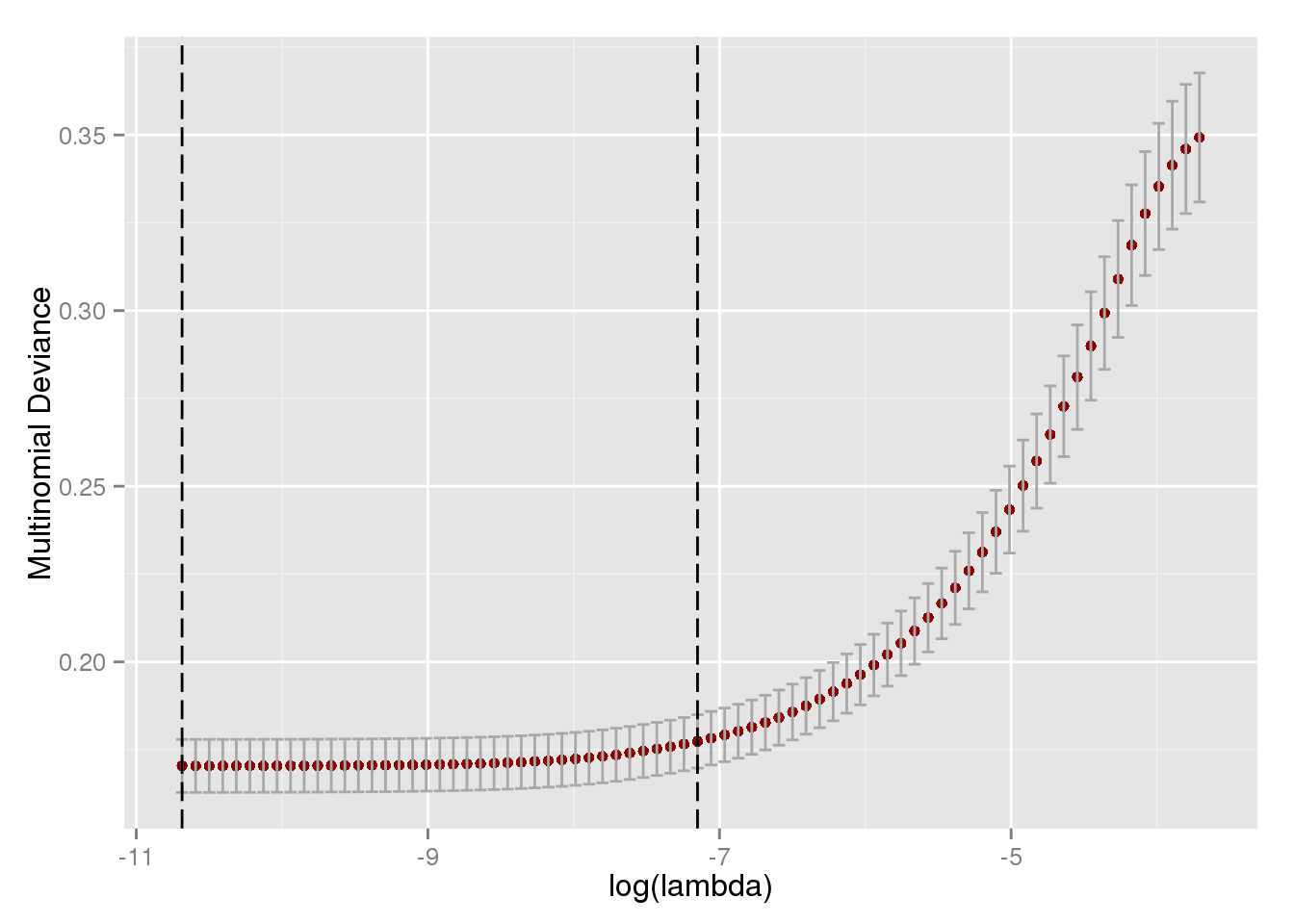As with the ridge model, the lasso model performs best with minimal shrinkage, though the fit worsens more slowly than in the ridge model. lasso.pred <- factor(predict(seismic.lasso, newx = x.test, type = "class")) err.lasso <- confusionMatrix(lasso.pred, y.test) err.lasso$table
##               Reference
## Prediction     earthquake explosion quarry blast
##   earthquake         1705        19           42
##   explosion             1         2            0
##   quarry blast          3         0            0
err.lasso$overall["Accuracy"] ## Accuracy ## 0.9633183 In contrast to the ridge model, however, the lasso model does make some attempt at distinguishing events, correctly identifying two explosions. Unfortunately, this comes at a cost of miss-classifying four of the earthquakes. ### Elastic Net Neither of the extremes of the regularization methods worked particularly well, notice that the ridge penalty lead to all earthquake predictions and the lasso penalty to miss-classifying more earthquakes than properly identifying explosions or quarry blasts. A compromise between the lasso and ridge models may strike a decent compromise. Because we now have two hyperparameters to optimize, we need to look beyond cv.glmnet() to fit the model and turn to the train() function from the caret package. Because the train object isn’t compatible with some of the other prediction and plotting functions already used, we’ll need to refit the model using glmnet. e.grid <- expand.grid(.alpha = (1:10)*(0.1), .lambda = 20^(-5:1)) seeds <- lapply(1:50, function(i) sample.int(1000, 10)) seeds <- append(seeds, sample.int(1000, 1)) cntrl <- trainControl(method = "adaptive_cv", repeats = 5, seeds = seeds, allowParallel = TRUE) seismic.train <- train(x.train, y = y.train, method = "glmnet", family = "multinomial", tuneGrid = e.grid, trControl = cntrl) (params <- seismic.train$bestTune)
##   alpha lambda
## 9   0.2 0.0025
seismic.enet <- cv.glmnet(x.train, y.train, family = "multinomial",
alpha = params["alpha"], parallel = TRUE)
ggnet(seismic.enet)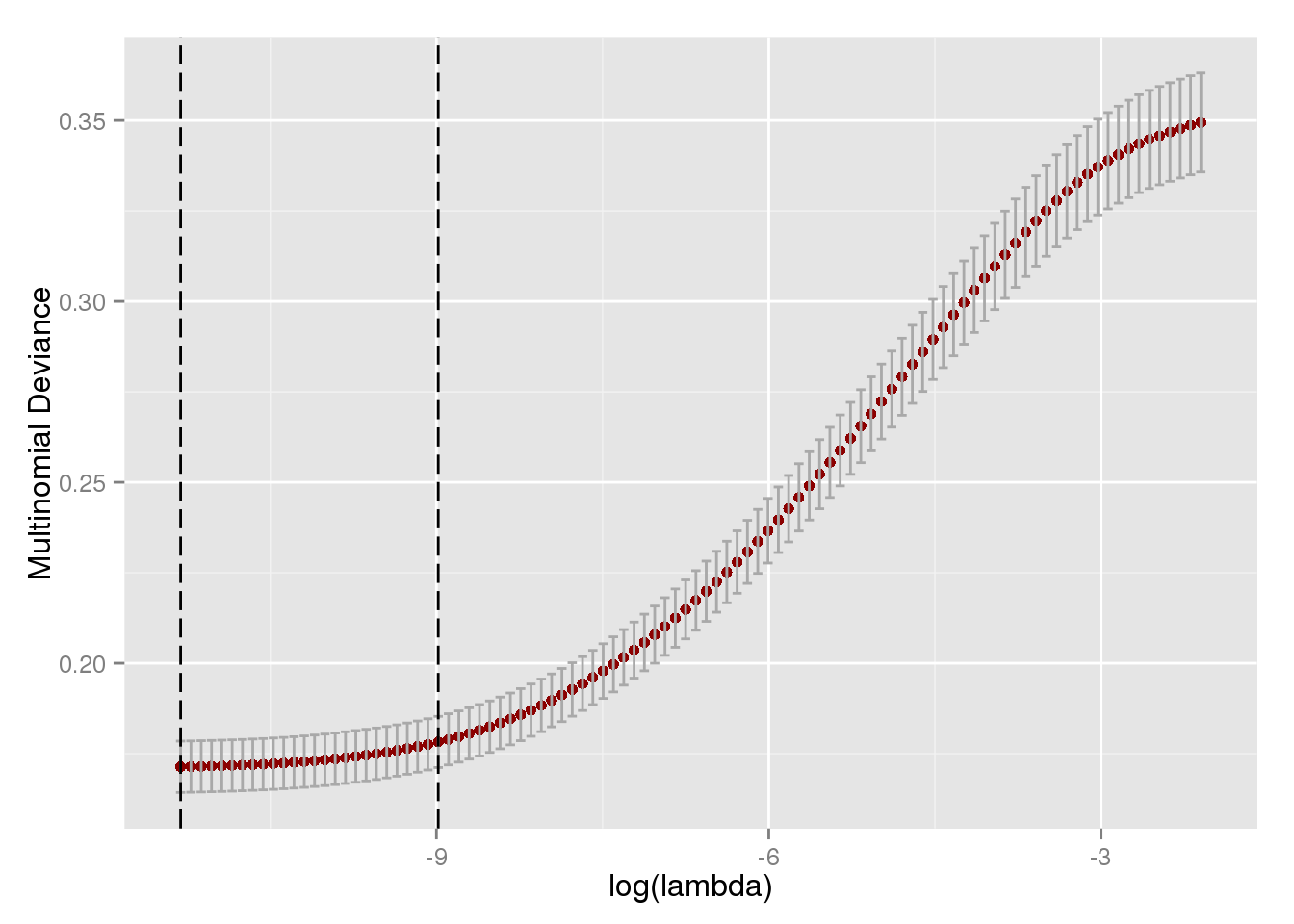So with our mixing parameter, we’re splitting the difference between ridge regression and the lasso.

enet.pred <- factor(predict(seismic.enet, newx = x.test, type = "class"))
err.enet <- confusionMatrix(enet.pred, y.test)
err.enet$table ## Reference ## Prediction earthquake explosion quarry blast ## earthquake 1705 19 42 ## explosion 1 2 0 ## quarry blast 3 0 0 err.enet$overall["Accuracy"]
##  Accuracy
## 0.9633183

Unfortunately, we see the same results as in the lasso. We do manage to correctly predict two explosions, but at the cost of missing the classification of four earthquakes, leaving us with a lower accuracy rate than ridge regression.

## Random Forest

library(randomForest)
## randomForest 4.6-10
## Type rfNews() to see new features/changes/bug fixes.
##
## Attaching package: 'randomForest'
##
## The following object is masked from 'package:dplyr':
##
##     combine
seismic.rf <- randomForest(x.train, y.train)
plot(seismic.rf, main = "Classification Error vs. Number of Trees")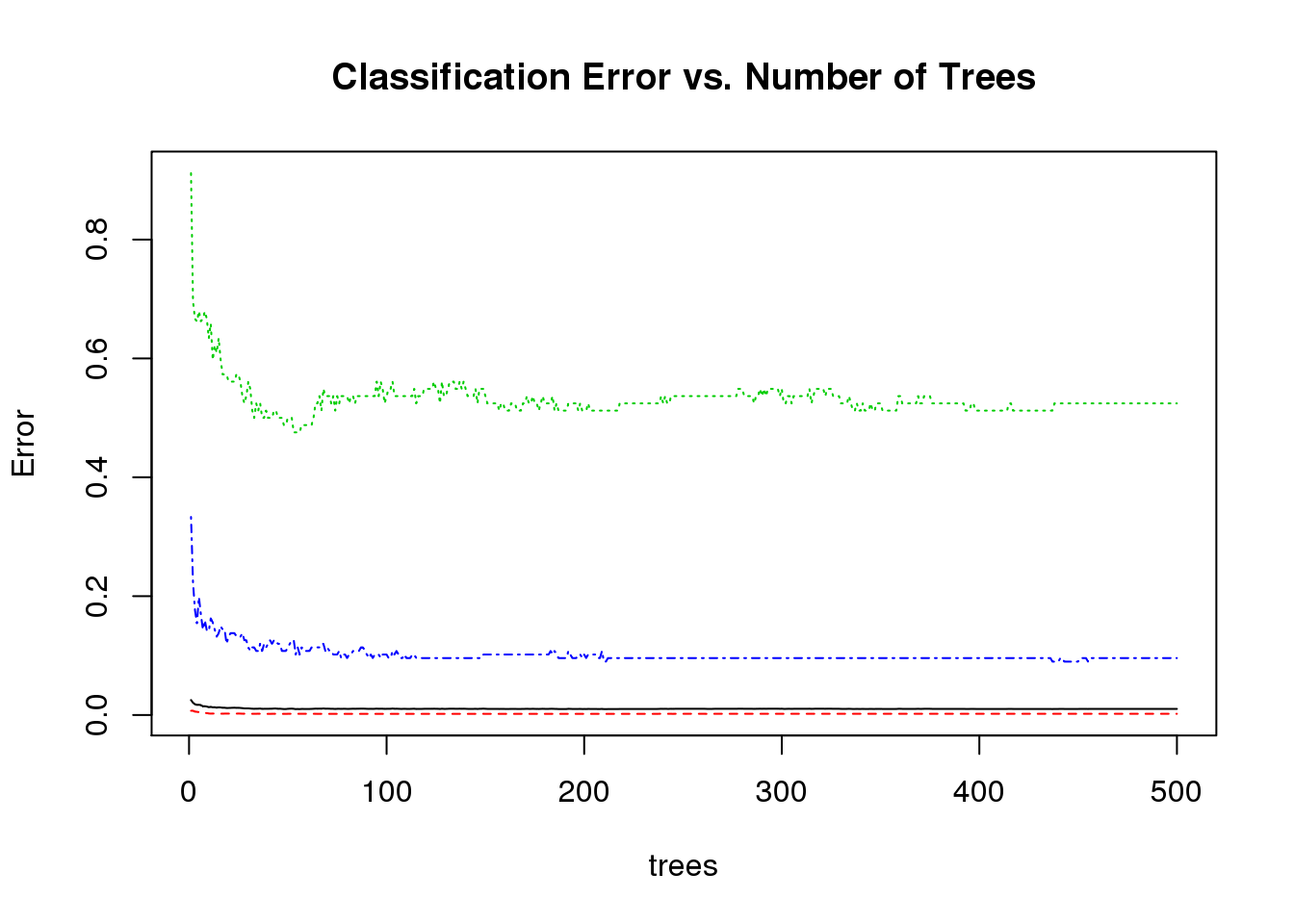The random forest has the most trouble classifying quarry blasts (the green line in the classification error plot), doesn’t a decent job with explosions, and performs quite well with earthquakes.

rf.pred <- predict(seismic.rf, newdata = seismic.test, type = "class")
err.rf <- confusionMatrix(rf.pred, y.test)
err.rf$table ## Reference ## Prediction earthquake explosion quarry blast ## earthquake 1703 0 3 ## explosion 4 11 2 ## quarry blast 2 10 37 err.rf$overall["Accuracy"]
## Accuracy
## 0.988149

While we do see some miss-classifying of earthquakes, the random forest does quite well overall. Thirty-seven of forty-two quarry blasts are correctly identified, but about half of all explosions are miss-classified.

Unlike the other models, however, the miss-classification of explosions is restricted to quarry blasts instead of earthquakes.

The model boasts and accuracy rate of $$98.81\%$$, a great improvement over the other techniques.

## $$k-$$ Nearest Neighbors

library(class)
knn.params <- tune.knn(x.train, y.train, k = 1:30, l = 0,
tune.control(sampling = "fix"),
validation.x = x.test,
validation.y = y.test)$best.parameters knn.pred <- knn(x.train, x.test, y.train, k = knn.params) err.knn <- confusionMatrix(knn.pred, y.test) err.knn$table
##               Reference
## Prediction     earthquake explosion quarry blast
##   earthquake         1706        20           27
##   explosion             0         0            0
##   quarry blast          3         1           15
err.knn$overall["Accuracy"] ## Accuracy ## 0.971219 Earthquakes were classified fairly well, with only three misses. Classifying explosions was less than successful, with twenty of the twenty one being misidentified as earthquakes Quarry blasts fared better than the explosions, but twenty-seven events of the forty-two were still classified as earthquakes. While the performance of $$k-$$NN is not at the same level as the random forest, we do better than the regularization based methods and the multinomial logit. ## Support Vector Machine svm.params <- tune.svm(x.train, y.train, tune.control(sampling = "fix"), validation.x = x.test, validation.y = y.test, gamma = 10^(-7:-1), cost = 10^(-1:1))$best.parameters
seismic.svm <- svm(x.train, y.train,
gamma = svm.params,
cost = svm.params)
svm.pred <- predict(seismic.svm, x.test)
err.svm <- confusionMatrix(svm.pred, y.test)
err.svm$table ## Reference ## Prediction earthquake explosion quarry blast ## earthquake 1709 21 42 ## explosion 0 0 0 ## quarry blast 0 0 0 err.svm$overall["Accuracy"]
## Accuracy
## 0.964447

As with the multinomial ridge model, the best the support vector machine could do with this data as simply classifying everything as an earthquake.

# Conclusions

Overall, this we see that his is a difficult classification problem because of the huge imbalance in the counts of our event types.

The simpler models, like $$k-$$ NN, the random forest, and the multinomial logit model, tend to perform better than the more complex fits.

Of course, this was just meant as a chance to practice these models and work on a fun problem. As I get the chance, I plan on exploring weighting methods for the observations and spatial models.